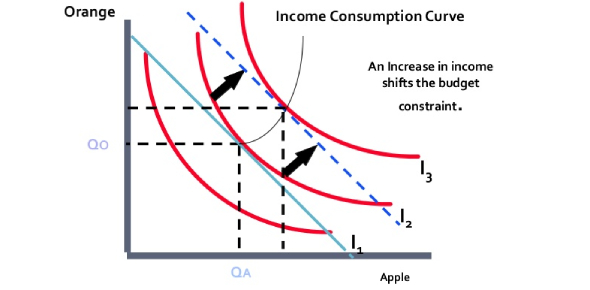# A Consumer Choice In Microeconomics Quiz

17 Questions | Attempts: 2027SettingsA Consumer Choice In Microeconomics Quiz; Microeconomics is a branch of economics that looks at how to allocate resources that are scarcer than others. It forces a firm or individual to think strategically and efficiently so that nothing goes to waste. In the following quiz, we’ll be taking a look at the subject of consumer choice within the overarching study of microeconomics and seeing just how much you can tell us about it.

• 1.
If we measure the quantity of French fries on the horizontal axis and the quantity of hamburgers on the vertical axis, and if the price of French fries is \$0.60 and the price of a hamburger is \$2.40, then the slope of the budget constraint is 1/4 (and it is negative).
• A.

True

• B.

False

• 2.
A budget constraint is a set of commodity bundles that provide the consumer with the same level of satisfaction
• A.

True

• B.

False

• 3.
Indifference curves measure the consumer's willingness to trade one good for another good while maintaining a constant level of satisfaction
• A.

True

• B.

False

• 4.
When drawn on a graph that measures the quantity of a good on each axis, indifference curves are usually straight lines that slope downward (negatively).
• A.

True

• B.

False

• 5.
Indifference curves tend to be bowed inward because a consumer is willing to trade a greater amount of a good for another if they have an abundance of the good they are trading away
• A.

True

• B.

False

• 6.
The limit on the consumption bundles that a consumer can afford is known as
• A.

An indifference curve

• B.

The marginal rate of substitution

• C.

The budget constraint

• D.

The consumption limit

• 7.
Suppose a consumer must choose between the consumption of sandwiches and pizza.  If we measure the quantity of pizza on the horizontal axis and the quantity of sandwiches on the vertical axis, and if the price of pizza is \$10 and the price of a sandwich is \$5, then the slope of the budget constraint is
• A.

5

• B.

10

• C.

2

• D.

1/2

• 8.
The slope at any point on an indifference curve is known as
• A.

• B.

The marginal rate of substitution

• C.

• D.

The marginal rate of indifference

• 9.
Which of the following statements is not true with regard to the standard properties of indifference curves?
• A.

Indifference curves are downward sloping

• B.

Indifference curves do not cross each other

• C.

Higher indifference curves are preferred to lower ones

• D.

Indifference curves are bowed outward

• 10.
The consumer's optimal purchase of any two goods is the point where
• A.

The consumer reaches the highest indifference curve subject to remaining on the budget constraint

• B.

The consumer has reached the highest indifference curve

• C.

The two highest indifference curves cross

• D.

The budget constraint crosses the indifference curve

• 11.
Which of the following is true about the consumer's optimum consumption bundle? At the optimum,
• A.

The indifference curve is tangent to the budget constraint

• B.

The slope of the indifference curve equals the slope of the budget constraint

• C.

The relative prices of the two goods equals the marginal rate of substitution

• D.

All of the above are true

• E.

None of the above are true

• 12.
Suppose we measure the quantity of good X on the horizontal axis and the quantity of good Y on the vertical axis.  If indifference curves are bowed inward, as we move from having an abundance of good X to having an abundance of good Y, the marginal rate of substitution of good Y for good X (the slope of the indifference curve)
• A.

Rises

• B.

Falls

• C.

Stays the same

• D.

Could rise or fall depending on the relative prices of the two goods

• 13.
If an increase in a consumer's income causes the consumer to increase his quantity demanded of a good, then the good is
• A.

An inferior good

• B.

A normal good

• C.

A substitute good

• D.

A complementary good

• 14.
If an increase in a consumer's income causes the consumer to decrease her quantity demanded of a good, then the good is
• A.

An inferior good

• B.

A normal good

• C.

A substitute good

• D.

A complementary good

• 15.
If an increase in a consumer's income causes the consumer to increase her quantity demanded of a good, then the good is
• A.

An inferior good

• B.

A normal good

• C.

A substitute good

• D.

A complementary good

• 16.
If income and prices were both to double, the budget line would
• A.

Shift outward in a parallel fashion

• B.

Shift inward in a parallel fashion

• C.

Stay the same

• D.

Rotate inward

• E.

Rotate outward

• 17.
Which of the following is not true regarding the outcome of a consumer's optimization process?
• A.

The consumer has reached his highest indifference curves subject to his budget constraint

• B.

The marginal utility per dollar spent on each good is the same

• C.

The consumer is indifferent between any two points on his budget constraint

• D.

The marginal rate of substitution between goods is equal to the ratio of the prices between goods

• E.

The consumer's indifference curve is tangent to his budget constraint

## Related TopicsBack to top
×

Wait!
Here's an interesting quiz for you.